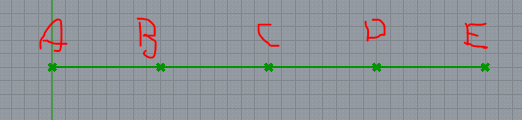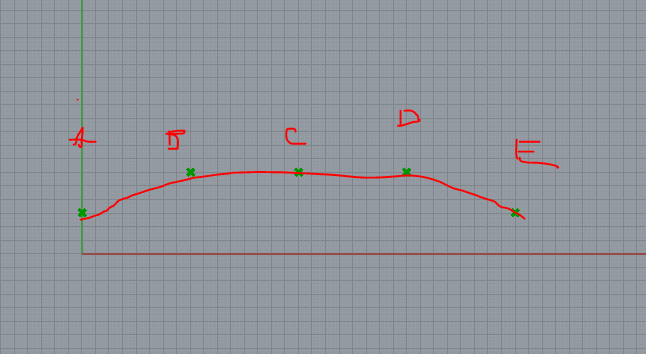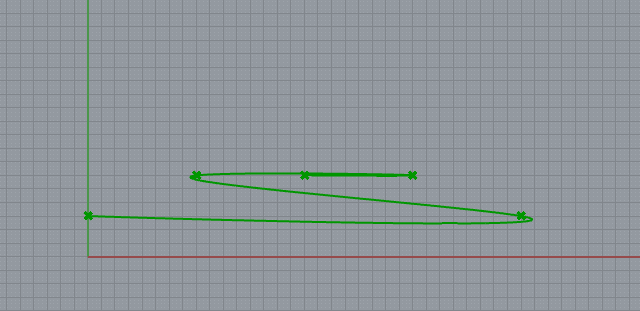# Re-organizing list of points/creating control points

After dividing a curve, i am controling each points and trying to reconstruct the curve through the points. In this example the curve is devided into 4 parts giving me 5 points.For my purposes, my script is written in such a way point (A and E) and points (B and D) move the same distance creating a symetrical curve. The resulting curve i am trying to obtain is something like this:But the points are out of order and hence get something like this while trying to reconstruct the line:What would be the simplest way to acheive this? Is there a way to convert these points into control points for the original curve so i wont have to reconstruct it?
control points on curve.gh (9.5 KB)

Since the input in merge starts with point A and E, the order is changing.
One way could be to replace the items:

control points on curve_DS.gh (13.5 KB)
I think there can be other methogs that might work for you.

1 Like

This works well! To make it even more convenient the next step could be to somehow automate this so that dividing the curve into even numbers directly gives you symmetrical control points you can control freely without having to re-do this process.

if points will always move symmetrically, like [0, n], [1, n-1] and so on, then I’d do something like this:

control points on curve_Re.gh (14.0 KB)

the main point is to not change the order of the points by isolating them with List Item (and selecting them in couples) but just to move them with a matching structure of vectors

but keep in mind that this works for a number of points up to 10 (which is the maximum value that was set on the division Count slider)
if you raise that number over 10, you’ll need to manually add more sliders to the lower Merge component

1 Like

This is super convinient, Thank you